# STEM Disease Models

New models are continually being added to STEM. Today STEM comes with a variety of basic models which implement algorithms such as those found in RM Anderson & RM May's textbook on Infectious Diseases of Humans: Dynamics and Control, Oxford and New York: Oxford University Press, 1991. ISBN 0198545991. STEM also includes more advanced models created by groups investigating current problems in epidemiology. As a framework users are also encouraged to create their own models and to even contribute them back to STEM.

# Basic Models

STEM comes with implementations of many standard epidemiological compartment models. These include both Stochastic and Deterministic SI, SIR, and SEIR models as well as models with seasonal forcing and nonlinear interaction terms.

## SI and SIS models

The simple two-state SI or SI(S) model is useful in describing some classes of microparasitic infections to which individuals never acquire a long lasting immunity. Certain RNA viruses such as rhinoviruses and coronaviruses (the common cold) mutate so rapidly that individuals recently recovered from a cold will still be susceptible to other strains of the same virus circulating in a population. In a simple model for this process, individuals never enter a recovered state, but rather alternate between being susceptible and being infectious.

## SIR and SIRS models

More generally, after exposure to microparasitic infection, individuals who recover from a disease will enter a third state where they are immune to subsequent infection. This Recovered State, R, appears in the SIR(S) compartment models. For infections that confer lifelong immunity in the recovered state, an SIR model is appropriate. Typical examples for which an SIR model is used include Paramyxovirus (measles) and Viral Parotitis (mumps). In cases involving the Orthomyxoviridae viruses (which cause seasonal flu), immunity is not lifelong and may decrease over time. Immunity loss can reflect a decrease in an individual’s immune response, or a genetic drift in the circulating strain of virus that diminishes the effectiveness of the acquired immunity. In either case, an SIRS model represents the rate at which people in a Recovered state return to a susceptible state.

## SEIR and SEIRS models

Some infectious diseases are also characterized by an incubation period between exposure to the pathogen and the development of clinical symptoms. If the exposed individual is not infectious during this incubation period (e.g., not shedding virus), it is important to model the incubation time explicitly. Note that there is a difference between an incubation time and a period of latency. A virus may or may not be dormant when an individual is in an exposed state. It is important to model the exposed (E) state explicitly when there is a delay between the time at which an individual is infected and the time at which that individual becomes infectious. In this case an SEIR(S) model is appropriate. Smallpox, for example, has an incubation period of 7-14 days

The disease models in STEM are implementations of these compartment models expressed as differential equations. These differential equations have a variety of parameters that are similar to the constants in a chemical rate equation. Users can easily change the basic parameters of any model in STEM with a text editor (called the property editor). This tunes the model based on the particular disease of interest. New models with even more advanced mathematics can easily be added to STEM - but that does take some knowledge of the Java programming language and of Eclipse. Please see the Tutorials and User Guides for detailed information on how to do this.

Once you have put together a model with a geographic region and other data (like transportation, time, and human population) you can easily export your work and share it with other users of STEM. In this way STEM is intended to promote scientific collaboration allowing researchers to build on each others work. See the section on Importing and Exporting Projects for instructions on how to do this and for information on some example scenarios.

## MacDonald Ross Disease Model

Our model for malaria transmission is based on work originally carried out by Ross (1911) and MacDonald (1957). Details can be retrieved from hereMacDonald Ross Disease Model.

Our model for malaria transmission is based on work originally carried out by Ross (1911) and MacDonald (1957). The cycle of the malaria and its transmission between secondary human hosts and primary vectors of the genus Anopheles is complex. It suffices to say that a human infection begins when sporozoites are injected by an infected female mosquito into the blood stream. The sporozoites migrate to the liver, and after some period of time they enter the bloodstream in the form of gametocytes which can be injected into a mosquito when it ingests human blood. Through a series of development within the mosquito, the injected gametocytes becomes gametes which (in the sexual phase) turns into a zygote, then a motile ookinete which bores through the gut of the mosquito and releases a large number of sporozoites. This completes the cycle.

Our model can be described by a set of differential equations describing the dynamics of the disease in both the human and the Anopheles vector. From the description of the malaria cycle above, it is clear that we need to model some period of latency from the time of the blood meal to the infectious stage in both human and vector. For humans, the latent period is defined as the time from initial infection to the appearance of gametocytes in the blood. For the Anopheles vector, the latent period is defined as the time from initial infection to the appearance of sporozoites in the mosquito saliva glands (also called sporogonic cycle). Once the Anopheles mosquito is infectious, it is assumed to stay infectious for the rest of its life. Humans, on the other hand, are able to clear gametocytes from the blood stream over time and do not stay infectious indefinitely. Once recovered from an infection, a human has built up antibodies against the parasite. However, antibodies decay over time and long periods of no exposure tend to result in lowered antibody titres within individuals.

The set of differential equations determining the state of a human population for a single region r is defined as:

where (leaving out the region) s(t), e(t), i(t) and r(t) are the relative number of susceptible, exposed, infectious and recovered humans, a is the biting rate on humans by a single mosquito (defined as the number of bites per unit of time), b is the fraction of infectious bites on humans that produces an infection,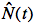is the total number of mosquitoes at time t (from our Anopheles model described earlier), N is the total number of humans (assumed constant over time),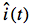is the relative number of infectious mosquitoes,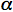is the immunity loss rate,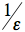is the human latent period and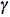is the rate at which humans recover from an infection.

The corresponding set of differential equations for the Anopheles mosquito population has fewer variables (since the mosquitoes never recover from an infection):

where (again leaving out the region),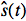,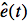and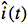are the relative number of susceptible, exposed and infectious mosquitoes, a is the biting rate (same as above), c is the fraction of bites by susceptible mosquitoes on infected people that produces an infection,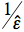is the latent period for the mosquito,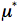is the background mosquito birth rate and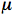is the background mosquito death rate.

The background mosquito birth rate () and death rate () deserve further explanation. The Anopheles model we described earlier determines the number of female mosquitoes in a region at any given time.depends on the four environmental factors as well as the human population density, and varies over time. However, whenremains constant, the background birth rate and death rate is for the mosquito is assumed the same (=) and it is described by a single parameter of the model. When the mosquito population is growing, we simply increase the birth rateto reflect the growth. When the population is shrinking, we reduce the birth rate. In some cases however, the mosquito population is shrinking so rapidly that setting the birth rate to zero is not enough. When this happens, we increase the death rateto keep up with the drop in the mosquito population.

Ross R (1911) The prevention of malaria (2nd ed.). Murray, London

Macdonald G (1957) The epidemiology and control of malaria. Oxford University Press, London

## Full Dengue Disease Transmission Model

We created a so called "full model" in order to capture a comprehensive picture of dengue transmission between host (human) and vector (mosquito) populations in STEM. Details can be obtained on the introductory page Dengue Disease Transmission Model.

## Nonlinear Models

STEM also implements nonlinear versions of the basic compartment models where the bilinear transmission kinetics term (bSI) is replaced with a modle of nonlinear transmission (e.g., bSIν, ν > 1 (see Liu, W-M., Hethcote, H. W. and S. A. Levin. 1987. Dynamical behavior of epidemiological models with nonlinear incidence rates. J. Math. Biol. 25: 359-380). Nonlinear models can be used to model saturation or " swamping " of the immune system as a function of disease burden or repeated exposure.

## Seasonal Tranmission (Forcing Function) Models

### Sinusoidal Model

Many diseases exhibit periodic or quasi-periodic epidemics, the most well being seasonal influenza. Recent experiments using guinea pigs suggest that seasonal variations in influenza incidence may be explained by small changes in transmission rate due to changes in temperature and relative humidity (Lowen et al., 2007; Shaman et al., 2009). Since SIR(S) disease models have an intrinsic tendency to oscillate (although with dampened effect), such changes can be further amplified by dynamic resonance caused by the population dynamics of the host-pathogen system (Dushoff et al., 2004).
As defined by Dushoff (2004), a SIR(S) model has a natural frequency

where γ is the recovery rate, α the immunity loss rate, and Ro the basic reproductive number. While a deterministic SIR(S) model with constant transmission will not exhibit sustained oscillations, it can demonstrate “damped” oscillations at this natural frequency, with the epidemic eventually dying out or tending to a fixed point of endemic infection. If the transmission rate includes a periodic excitation term (a forcing function) tuned to the same resonant frequency, seasonal oscillations may be sustained. If the frequencies differ, as is the case for any forced harmonic oscillator, the system may exhibit sustained oscillations with “beats” resulting in some epidemics that are larger than others.

STEM includes advanced models seasonally modulated transmission coefficient β(t). A deterministic SIR(S) model with constant transmission will never produce sustained periodic epidemics or seasonality (Dushoff et al., 2004). The simplest seasonal transmission function implemented in STEM uses periodic forcing function:

β(t) = βo[(1 - x) + x sin(ωt + φ)]

where βo is the(max) transmission amplitude and x determines the periodic part of the excitation function (0<=x<=1).

### Gaussian Model

We are also developing a periodic excitation function based on a linear combination of (periodic) gaussian excitations. This function may be useful in modeling data where the size of the epidemic varies year to year based on known differences in transmissibility of a disease (for example variations in the dominant serotype or strain).

We define β(t) as:

where i is the index of the yearly influenza season; βi is the peak transmission coefficient for season i; (1-ρi) is the fraction by which transmission varies seasonally in season i (0 < ρi < 1.0); ti is the day of peak transmission for season i; g(x, σi) is the probability distribution function of a Gaussian distribution with mean 0 and standard deviation σi; and ξ(t,ti) is the mixing function. The day of peak transmission, ti, is defined so that the transmission function has a period of exactly 1 year, with constant phase, φ. That is transmission peaks φ days after January 1 of season i. The joining function, ξ(t,ti), smoothly joins adjacent Gaussians so that β(t) is only dependent upon a single Gaussian during the period of season i where most transmission occurs

### Interpolation of Sampling Points

The seasonal variations of the incidence of a disease can be caused by different things. Climate changes can be the reason as mentioned above, but even the beginning and the end of the school year can have an influence on the disease. In order to be able to model all kinds of seasonal variations, the STEM user should be able to specify every possible excitation function, which can be done as shown in the following. The user specifies the transmission rate for several days in the year and interpolation is used to compute the transmission rate for all remaining days. The simplest interpolation method is linear interpolation. To get a smooth function spline interpolation can be used.

# Models of Population

## Basic Models

### Standard Population Model

The standard population model can be used to model a population that growths or shrinks by a background birth rate and death rate. It is commonly used to model basic human population dynamics.

### Mosquito Population Model

The mosquito population model in STEM uses environmental earth science data (available in the STEM library) to estimate the average mosquito population for a region. Our goal is to compute temporal and regional probability for presence of mosquitoes from environmental variables. If we assume that the mosquito probability at time t and location r follows a Poisson distribution, and if the density depends on independent variables describing or defining the local environment at (t,r), then the logarithm of the expected value for the (un-normalized) probability can be expressed as a linear combination of the independent environmental variables (Charoenpanyanet 2009, Cameron 1998, Christensen 1997):

P(t, r) = kP(T, t, r)*P(R, t, r)*P(V, t, r)*P(E, r) (Equation 1)

Suppressing the space and time variables (t,r), P(T) represents the temperature dependence, P(R) represents the rainfall dependence, P(V) represents the NDVI dependence and P(E) the elevation dependence. If any of functions is zero, then the joint probability is also zero. By expressing the total risk as a joint probability distribution based on a product of environmental functions, we can normalize or rescale the expected mosquito probability using a single population calibration constant k. This calibration constant is chosen such that the value of P is calibrated to the observed mosquito burden.

In Equation 1, the temperature factor P(T) uses average night time surface temperature (Celsius). Daily mosquito survival p (assuming constant humidity) is defined as (Martens 1997):

Another temperature dependent variable is the larval duration ld (Jepson 1947):

We define our temperature factor P(t) as the combined effect of ld and p (Craig 1999):

P(T) = (-4.4+1.31T-0.03T^2)*(0.00554T-0.06737) (Equation 4)

If the temperature is less than 16 C or greater than 40 C, we set P(T) to zero. This figure shows a plot of P(T) versus temperature T:

The elevation factor in Equation 1 uses altitude above sea level in meters. Field studies of Anopheles in Thailand along with remotely sensed elevation data in both wet and dry seasons suggest Anopheles population size is strongly peaked at an elevation of ~400 meters (Charoenpanyanet 2009). We parameterize the Thailand observations with an elevation function that increases linearly from 0-400 meters, and falls linearly from 400-1200 meters, and is zero above 1200 meters.

The dependency on rainfall P(R) is based on mosquito density surveys. In a rural town in western Kenya, the average number of blood fed An. Gambiae and funestus mosquitos per house was measured (per month) between October and May 2000 (Okara 2010). The data suggest an approximately linear relationship between mosquito population and rainfall level. Hydrologic studies suggest rainfall leads to increases in standing water necessary for larval growth. We model P(R) with a simple linear approximation:

P(R) = R(t, r) (Equation 6)

where R(t, r) is the monthly average rainfall (mm) at location r and time t.

The vegetation factor P(V) is based on the Normalized Difference Vegetation Index (NDVI). The NDVI algorithm used in the TERRA/MODIS mission is described in detail by (Huete et al. 1999). The NDVI scale ranges between -0.1 and 0.9 where -0.1 represent bodies of water; values close to 0 correspond to sand, rock, desert or snow; and values between 0.2 and 0.4 represent shrubs and grassland. NDVI near 0.8-0.9 corresponds to tropical rainforests or other forested areas with high density of green leaves. NDVI changes seasonally and over longer time scales with global climate change and deforestation.

A field survey of 26 sites in Thailand measured the dependence of mosquito density on NDVI, as determined by the number of female mosquito bites per person-hour (Charoenpanyanet 2009). A survey in South Korea showed the effect of NVDI on the local density of larvae (Rueda 2010). In both studies, the mosquito population was found to be approximately zero below NDVI <0.3, and to increase approximately linearly for NDVI > 0.3. Based on these works we approximate the vegetation factor as:

Our model for Malaria transmission (see below) depends on an estimate of the actual number of mosquitoes in a region. We assume that female Anopheles mosquitoes feed exclusively on humans (no malaria reserve is assumed in animal populations). So if no humans inhabit an area, no mosquitoes can survive. Furthermore, we assume a mosquito travels at most 50 meters in search of a blood meal, so if the human areal density is less than 127 people/km2 (1 person in a 50 meter radius circle), mosquito survivability is reduced.

Our estimate for the number of (female) mosquitoes in a region, N(t, r) , is:

where A(r) is the area (in km^2) for region r,is the human population density of region (in humans/ km^2). Observe that in this case, the scaling factor k in Equation 1 is calibrated to observed mosquito areal density.

Charoenpanyanet A (2009) Anopheles Mosquito Density Predictive Model Based on Remotely Sensed Data. Doctoral Dissertation Asian Institute of Technology, Bangkok, Thailand

Cameron AC, Trivedi PK (1998) Regression Analysis of Count Data. New York: Cambridge University Press.

Christensen R 1997 Log-Linear Models and Logistic Regression, 2nd edn. New York: Springer-Verlag.

Martens WJM, Jetten TH, Rotmans J, Niessen LW (1995) Climate change and vector-borne diseases. Global Environmental Change, Vol 5. No. 3. pp. 195-209, 0959-3780(95) 00051-8

Jepson WF, Moutia A, Courtois C (1947) The malaria problem in Mauritius: the bionomics of Mauritian anophelines. Bull. Entomol., Res. 34, 89

Craig MH, Snow RW, le Seue D (1999) A climate-based sistribution model of malaria transmission in Sub-Saharan Africa. Parasitology Today, vol. 15, no. 3.

Huete A, Justice C (1999) MODIS VEGETATION INDEX (MOD 13) Algorithm theoretical basis document (http://modis.gsfc.nasa.gov/data/atbd/atbd_mod13.pdf)

Rueda LM, Brown TL, Kim HC, Chong ST, Klein TA, Foley DH, Anyamba A, Smith M, Pak EP, Wilkerson RC (2010) Species composition, larval habitats, seasonal occurrence and distribution of potential malaria vectors and associated species of Anopheles (Diptera: Culicidae) from the Republic of Korea. Malaria J 9:55. doi:10.1186/1475-2875-9-55.

## Multipopulation Models

### Demographic Population Model

The Demographic Population Model allows to divide the whole population into groups. This can be useful to model disease it must be distinguished between men and women, children and adults etc.. The model is based on the Standard Population Model, so the population growths or shrinks by a background birth rate and death rate.

### Aging Population Model

This model is an enhancement of the Demographic Population Model. It allows to divide the population into groups regarding the people's age. The process of aging is also implemented, which means that fractions of each age group move to the respective next age group as time passes. Additional documentation may be bound on the tutorial page Using Aging Populations in STEM.